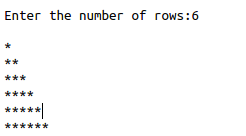• #5, First Floor, 4th Street Dr. Subbarayan Nagar Kodambakkam, Chennai-600 024 Landmark : Samiyar Madam
• pro@slogix.in
• +91- 81240 01111

### What is Range and Recursive function in python?

###### Description

To see what is range() function and recursion in python and its important uses.

#### Range().

It generates sequence of numbers in a given interval.

It starts its index position as zero, unless index position is declared explicitly.

Eg.>>>range(5)

Above code produce 0,1,2,3,4

Its very useful for generating loop sequence like pattern programs, prime numbers ,etc..

#### Recursive function.

Which function is called by itself when the program is running, that is called recursive function.

Recursion works like a loop.

###### Sample Code

Source code(Floyd pattern):

#Get input from user

n = int(input

(“Enter the number of rows:”))

#make first loop sequence

for i in range(n+1):

#make second loop

for j in range(i):

#printing the pattern

print(“*”,end=””)

print()

Source code(Factorial program):

#recursive function

print(“Factorial using recursion******”)

def calc_factorial(x):

if(x==1):

return 1

else:

return(x*(calc_factorial(x-1)))

#get input from user

n=int(input(“Enter the number:”))

#method calling statements

print(“the factorial of given number is:”,

calc_factorial(n))

###### Screenshots